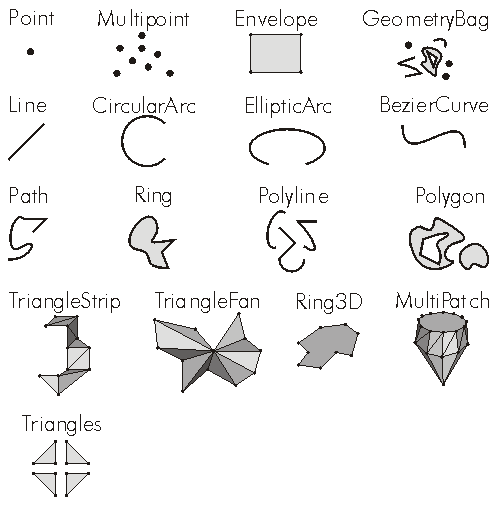ArcObjects Library Reference (Geometry)

# IGeometry.GeometryType Property

The type of this geometry.

```[Visual Basic .NET]
Public ReadOnly Property GeometryType As esriGeometryType```
```[C#]
public esriGeometryType GeometryType {get;}```
```[C++]
HRESULT get_GeometryType(
esriGeometryType* shapeType
);```
```[C++]
Parameters
shapeType [out, retval]
shapeType is a parameter of type esriGeometryType
```

#### Product Availability

Available with ArcGIS Engine, ArcGIS Desktop, and ArcGIS Server.

#### Remarks

`esriGeometryNull          = 0esriGeometryPoint         = 1esriGeometryMultipoint    = 2esriGeometryPolyline      = 3esriGeometryPolygon       = 4esriGeometryEnvelope      = 5esriGeometryPath          = 6esriGeometryAny           = 7esriGeometryMultiPatch    = 9esriGeometryRing          = 11esriGeometryLine          = 13esriGeometryCircularArc   = 14esriGeometryBezier3Curve  = 15esriGeometryEllipticArc   = 16esriGeometryBag           = 17esriGeometryTriangleStrip = 18esriGeometryTriangleFan   = 19esriGeometryRay           = 20esriGeometrySphere        = 21`#### See Also

IGeometry Interface

#### .NET Snippets

Add Graphic to Map | Flash Geometry

#### .NET Samples

3D multipatch examples (Code Files: GeometryUtilities) | Custom UI elements using add-ins (Code Files: AddGraphicsTool) | Bind a geodatabase table to a .NET control (Code Files: FieldPropertyDescriptor) | RSS weather layer (Code Files: RSSWeatherLayerClass) | Create a custom tool (Code Files: DrawGraphicLine)

#### .NET Related Topics

Casting between interfaces | Create a custom tool | How to use the IEnumSegment methods | Simplifying a feature geometry | Working with the edit sketch